Oscillator Suppression in the Blood Flow Regulation of Interacting, Non- Identical Nephrons | OMICS International
Journal of Hypertension: Open Access
All submissions of the EM system will be redirected to Online Manuscript Submission System. Authors are requested to submit articles directly to Online Manuscript Submission System of respective journal.

# Oscillator Suppression in the Blood Flow Regulation of Interacting, Non- Identical Nephrons

Yulia P Emelianova1*, Alexander P Kuznetsov2, Jakob L Laugesen3, Erik Mosekilde3 and Niels-Henrik Holstein-Rathlou4

1Institute of Electronics and Mechanical Engineering, Russia

3Department of Physics, Technical University of Denmark, Denmark

4Department of Biomedical Sciences, University of Copenhagen, Denmark

*Corresponding Author:
Yulia P Emelianova
Institute of Electronics and Mechanical Engineering
Yuri Gagarin State Technical University of Saratov
Polytechnicheskaya 77, 410054 Saratov, Russia
Tel: 79172021488
E-mail: [email protected]

Received Date: April 25, 2014; Accepted Date: May 28, 2014; Published Date: May 30, 2014

Citation: Emelianova YP, Kuznetsov AP, Laugesen JL, Mosekilde E, Holstein-Rathlou NH (2014) Oscillator Suppression in the Blood Flow Regulation of Interacting, Non-Identical Nephrons. J Hypertens 3:153. doi:10.4172/2167-1095.1000153

Copyright: © 2014 Emelianova YP, et al. This is an open-access article distributed under the terms of the Creative Commons Attribution License, which permits unrestricted use, distribution, and reproduction in any medium, provided the original author and source are credited.

Visit for more related articles at Journal of Hypertension: Open Access

#### Abstract

Background: Regulation of the blood flow to the individual functional unit (nephron) of the kidney involves a feedback mechanism that produces large-amplitude oscillations in the blood flow itself as well as in the intra-nephron pressures and flows. Neighboring nephrons adjust their blood flow variations relative to one another via signals that propagate along the interconnecting blood vessels.

Purpose and method: Using a detailed physiological-based model of a pair of vascular coupled, non-identical nephrons, the paper examines the effect that their relative oscillatory strength has on the behavior of the coupled system. This is of direct interest in connection with ongoing work attempting to study the synchronization behavior for larger groups of superficial nephrons by means of laser speckle contrast imaging.

Results: Our analysis demonstrates that a region of so-called “broadband Synchronization” may develop between those coupling strengths at which the stronger oscillating nephron starts to suppress the autonomous oscillations of the weaker nephron and those coupling strengths at which the two nephrons mutually inhibit each other’s oscillations. We suggest that the transition be-tween synchronized and suppressed dynamics may have a physiological significance comparable to the transition from ergodic to synchronized periodic dynamics.

#### Keywords

Synchronization; Oscillator suppression; Total oscillator death; Physiological regulation; Nephron auto regulation

#### Introduction

The transition to synchronization for two coupled autonomous oscillators is known to proceed along one of two possible routes [1,2]. In the regime of relatively low coupling, the asynchronous state (quasiperiodicity) can be depicted as an ergodic motion on the surface of a two-dimensional torus, and the transition to synchronization takes place via a saddle-node bifurcation that leads to a pair of stable and unstable resonance cycles on the torus surface. When varying the mistuning between the oscillators, this mechanism gives rise to a dense set of synchronization regions (Arnol’d tongues) organized in accordance with their so-called rotation number, i.e., the ratio of the periods for the uncoupled oscillators [3,4].

The second type of transition is commonly referred to as “suppression of the natural dynamics” [1,2]. For an externally forced oscillator, this transition implies that the torus, on which the forced oscillatory dynamics takes place, collapses and the system is left with a simple periodic motion representing the dynamics of its equilibrium point in the presence of the external forcing. Similarly, if the coupling is strong enough, a system of two non-identical oscillators may undergo a transition from (synchronized or ergodic) two-mode dynamics to a suppressed state in which the weaker oscillator no longer maintains its endogenous dynamics, but continuous to oscillate only under the forcing of the stronger oscillator.

In regions between the resonance tongues, the oscillator suppression mechanism involves an inverse torus-birth bifurcation (secondary Hopf bifurcation).In the resonance zones, the transition follows a number of generic rules [5,6] such that, for instance, the 1:1 tongue assumes a triangular (Vshaped) form and is terminated at high coupling strengths by a saddle-node bifurcation curve, the 1:2 tongue ends in a bubble of one or more closed period-doubling curves, and the 2:3 resonance tongue terminates with a loop of its delineating saddlenode bifurcation curve around the so-called period-3 resonance point on the torus bifurcation curve.

For even higher coupling strengths, a system of two coupled, nonidentical oscillators can undergo a second transition, now to the regime of “total oscillator death” [7,8]. This transition involves an inverse Hopf bifurcation in which the equilibrium point of the coupled system stabilizes, and the oscillatory dynamics comes to a complete stop as the stronger oscillator no longer is capable of driving the weaker oscillator.

It seems reasonable to expect that the transition between synchronized and suppressed oscillations can play a role in the regulation of physiological systems comparable, perhaps, even to that of the transition between quasi periodic and synchronized periodic oscillations. However, although they represent very different forms of dynamics, synchronized and suppressed oscillations are quite difficult to distinguish in experimental situations. Moreover, as model studies show, the region of suppressed oscillations often covers a significantly larger proportion of parameter space than do the synchronization regions. As a result, many cases of oscillator suppression may incorrectly be interpreted as representing cases of synchronization. A way to discriminate between the two types of dynamics is also required to ensure that biomedical synchronization experiments are performed at forcing amplitudes that are low enough for the endogenous dynamics to be maintained.

In a number of recent papers, Kuznetsov et al. , Kuznetsov and Roman , and Emel’yanova and Kuznetsov  have examined the two synchronization transitions for a pair of non-identical van der Pol oscillators with dissipative coupling and with a relatively large difference in oscillator strength. This has led to a more detailed understanding of the phenomena that can occur in the region of oscillator suppression. In particular it was shown how the region of oscillator suppression can stretch as a broad band across a wide range of mistuning, and how the width of this band increases with the difference in oscillator strength.

The purpose of the present paper is to examine how the same phenomena unfold in a physiology-based model of two interacting, non-identical functional units of the kidney. We first determine the distribution of quasi periodic, synchronized periodic, and suppressed modes in the parameter plane spanned by the coupling strength and by the mistuning between the two physiological oscillators and illustrate how difficult it is to distinguish between synchronized and suppressed modes on the basis of experimentally available information in the form of time and phase plots. We determine the coupling strength at which the transition to oscillator suppression occurs as a function of the mistuning and show, in accordance with the previously obtained results for coupled limit cycle oscillators [9-11], that a region of suppression can stretch across a very broad range of frequency mismatch. Finally, we determine the bifurcation structure associated with the transition from suppression to oscillator death.

#### Oscillatory Phenomena in Nephron Autoregulation

The kidneys play an important role in maintaining a suitable environment for the cells of the body. In particular, the kidneys control the proportion of various blood solutes, the extracellular fluid volume, and the blood pressure. Figure 1 provides an illustration of the main structure of the functional unit of the kidney with the glomerulus, the different tubular sections, and the macula densa region. A human kidney contains approximately one million such nephrons operating in parallel, and a rat kidney about 30000. For superficial nephrons, the proximal tubules are visible in the surface of the kidney. This allows relatively long time series (20-40 min) of the proximal tubular pressure to be obtained by means of a small pressure pipette. The flow of blood to the individual functional unit (nephron) of the kidney is regulated by two different mechanisms: (i) a myogenic mechanism that adjusts the flow resistance of the afferent arteriole in response to changes in the arterial blood pressure, and (ii) the so-called tubuloglomerular feedback (TGF) mechanism that responds to changes in the salt concentration of the tubular fluid near the terminal part of the loop of Henle. The TGF mechanism represents a negative feedback . However, due to a delay of approximately 15 s associated with the fluid flow through the tubular system, the feedback tends to be unstable and to produce large scale limit cycle oscillations with a period of about 30-40 s. The presence of such oscillations has repeatedly been demonstrated in experiments on rats [13,14]. It has also been shown that the oscillations tend to become chaotic in rats with elevated blood pressure [15,16]. For normotensive rats about half of the measured time series for the tubular pressure oscillations demonstrate episodes of period-2 dynamics with characteristic alternation between high and low maxima [17,18].

Figure 1: Sketch of the functional unit of the kidney with the glomerulus, the different tubular sections and the macula densa region. Note how the distal tubule passes within cellular distances of the afferent arteriole for the same nephron. This unusual physiological structure provides the basis for the TGF regulation.

The myogenic (or vasomotoric) mechanism also produces oscillations associated with periodic contractions of the smooth muscle cells around the afferent arteriole . For rat kidneys, the myogenic oscillations typically display a period of 6-8 s, or about five times shorter than the period of the TGF mediated oscillations. The myogenic oscillations are clearly detectable in the measured tubular pressure variations for both normotensive and hypertensive rats . The two regulatory mechanisms both work through activation of the smooth muscle cells in the afferent arteriolar wall. This provides for interaction between the two regulatory modes, and wavelet analyses of the experimental pressure variations have clearly revealed the occurrence of intra-nephron modes with 4:1, 5:1, and 6:1 synchronization .

Neighboring nephrons interact with one another both via the displacement of blood from one nephron to the other (so-called hemodynamic coupling) and via signals of muscular activation that travel from nephron to nephron along the blood vessels (vascular propagated coupling). These interactions tend to synchronize the tubular pressure oscillations in neighboring nephrons . Wavelet analyses of experimental time series have shown that both in-phase and anti-phase operation between neighboring nephrons occur.

The nephron model to be used as an example for our discussion of oscillator suppression and total oscillator death in a system of coupled; non identical biological integrates the most essential mechanisms of nephron autoregulation into a consistent and physiological realistic picture. Over the years, a number of different versions of the model have been developed [22-24], each emphasizing specific aspects of the problem such as the absorption of water and salts along the loop of Henle  or the signal transmission between the macula densa cells and the smooth muscle cells in the arteriolar wall . The present analysis is based on a version developed by Barfred et al. . Due to its relative simplicity, this version is particularly suited for detailed bifurcation studies, and the model has previously been used for studies of interacting nephrons [25,26].

Different models, including or excluding specific mechanisms, have been formulated by other groups. In particular Layton et al. [27,28] have developed a model of the TGF regulation and the transport of solutes along the ascending thick part of the loop of Henle to investigate potential sources of irregular pressure variations and explain the complex power spectra observed for hypertensive rats. However, excitation of high frequency overtones as suggested by the Layton model does not conform to the period-doubling processes revealed by the experimental time series and by the associated power spectra. Other models relating to the function of the individual nephron focus on the urine concentration mechanism in the rat outer medulla  or on the processes that occur in the distal tubule [30,31].

#### The Biophysical Oscillator Model

To illustrate the physiological significance of oscillator suppression and total oscillator death we shall consider a model of two interacting, non-identical nephrons. The description of the individual nephron focuses on the regulation of the incoming blood flow through cooperation between the tubuloglomerular feedback (TGF) mechanism and the myogenic (or vasomotoric) response. Both mechanisms act through adjustments of the afferent arterial flow resistance Ra. As described in the previous ection, the TGF mechanism reacts to changes in the salt concentration at the macula densa region near the end of the loop of Henle, and the faster myogenic mechanism responds to variations in the arterial blood pressure. The first component of our model is a conservation equation that relates changes in the proximal tubular pressure Pt to the rate of glomerular filtration Ffilt, the reabsorption of filtrate that takes place in the proximal tubule Freab, and the flow into the loop of Henle FHen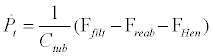(1)

Here, Ctub=3 nl/kPa denotes the elastic compliance of the tubule. As other parameters in the model, Ctub has been estimated from independent measurements [23,32]. Obviously, this involves a certain uncertainty stemming both from the representation of a distributed system by a lumped model and from the inhomogeneity of the nephron population. For simplicity, the rate of proximal tubular reabsorption Freab=0:3 nl/s is considered to be constant. The rate of filtration and the flow into the loop of Henle are given by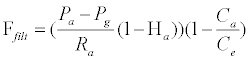(2)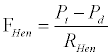(3)

where the glomerular pressure satisfies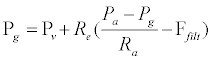(4)

and the protein concentration in the efferent blood is given by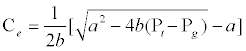(5)

Here, Pa=13:3 kPa denotes the arterial blood pressure. Ha=0:5 is the afferent hematocrit, and Ca = 54 g/l the afferent blood protein concentration. Pd=0:6 kPa is the distal tubular pressure, P_=1:3 kPa is the venous pressure, and RHen=5:3 kPa/(nl/s) is the flow resistance in the loop of Henle. Re=1:9 kPa/(nl/s) is the efferent arteriolar resistance, and a=21:7 Pa/(g/l) and b=0:39 Pa/(g/l)2 are parameters that relate protein osmotic pressure to protein concentration. Eqs. 2–5 directly express the assumptions that (i) protein is retained in the blood, (ii) the protein osmotic pressure at the efferent end of the glomerular capillaries balances the hydrostatic filtration pressure Pg-Pt, (iii) the flow into the loop of Henle is given by the pressure drop Pt-Pd divided by an effective flow resistance RHen, and (iv) the glomerular pressure may be determined by distributing the arterial to venous pressure drop between the afferent and efferent arteriolar flow resistances. The square root in Equation. 5 arises from an experimentally observed parabolic variation between protein concentration and protein osmotic pressure .

The delay in the flow of filtrate through the loop of Henle is modeled by means of the lag structure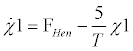(6a)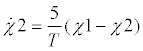(6b)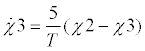(6c)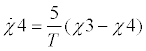(6d)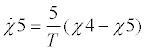(6e)

The delay time T will be used as a bifurcation parameter. The base case value is T = 14 s. In lack of detailed knowledge about the damping of the oscillations associated with the flow of fluid through the loop of Henle, previous versions of the nephron model applied a standard third order delay structure. This provided for a relatively high damping which in practice was compensated by increasing the gain factor of the TGF mechanism by about 50%. Use of a fifth order delay allows us to bring the gain factor into the physiological regime.

The steady state relationship between the pressure Peq across the arteriolar wall (the transmural pressure) and the normalized arteriolar radius r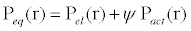(7)

is assumed to consist of a passive elastic component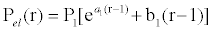(8)

in parallel with the active (muscular) component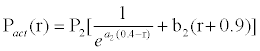(9)

Peq represents the transmural pressure at which the arteriolar wall is at equilibrium with its present radius and its present muscular activation. Expressions or Pel and Pact were obtained by Feldberg et al.  by integrating a simplified model of the strain-stress relationship across the arteriolar wall, while accounting for the fact that the muscular volume remains constant. The muscular tone expresses the degree of activation of the smooth muscle cells in the arteriolar wall. The parameters a1=10, a2=13, b1=36:4 and b2=1:53 were determined by fitting the above expressions Eqs 8 and 9 to available physiological data. The form of the active component represents the observation that activation of smooth muscle cells in the arteriolar wall only takes place in a range of radii around the resting state (r=1) for the vessel.

The TGF-mediated activation of the smooth muscle cells in the arteriolar wall can be determined by inserting a small lump of wax into the proximal tubule and measuring the rate of filtration (or the so-called stopflow pressure) as a function of the externally forced flow through the loop of Henle . This provides us with the open-loop feedback characteristics for the TGF mechanism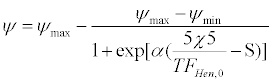(10)

As before, represents the muscular tone. The parameter determines the slope of the feedback characteristics. We will refer to as the gain factorfor the TGF mechanism. ¬5 is the delayed version of the flow into the loop of Henle provided by Eq. 6e. The parameters max=0:44, min=0:20, and S=1 - (1=) lnf (eq χ min)=(max χ eq) g with eq=0:35 are used to scale and position the feedback characteristic relative to the experimental results. Along with the time delay T, the feedback gain factor is considered as a bifurcation parameter. The base case value is=9. Excitation of vasomotoric oscillations in the (normalized) arteriolar radius is described by means of the externally driven second order differential equation.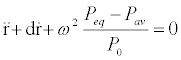(11)

Where the damping constant d=0:04 s-1. ω=s-1 represents a characteristic angular frequency and P0 = 20 kPa is a normalization pressure. The driving term for the myogenic oscillations is the difference between the equilibrium pressure Peq and the average blood pressure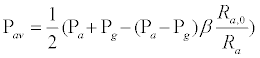(12)

in the active part of the afferent arteriole. The active part of the afferent arteriole is assumed to be the fraction (1) = 1=3 of the arteriole that is positioned next to the glomerulus. Excitation of the myogenic oscillations thus occurs in response to TGF-related variations in Peq and Pav. It is worth noting, however, that the model also functions for negative values of the damping constant d where the myogenic oscillations become self-sustained. If the second-derivative term ¨r in Equation. 11 is deleted, the myogenic oscillations disappear, but the model continues to function as a physiologically meaningful model of the TGF-mediated oscillations.

Finally, as derived from Poiseuille’s equation, the afferent arteriolar resistance is given by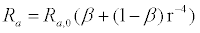(13)

where Ra,0=2:4 kPa/(nl/s) denotes the equilibrium value of the afferent arteriolar resistance. Note, that simulation of the model in the above form involves the solution of a third order algebraic equation in each integration step. Different approaches to this problem can be chosen. In the present paper, the explicit solution to the third order equation was used.

Figure 2a presents an overview of the mode distribution in the relevant part of parameter space. This allows us to follow the perioddoubling bifurcations and the transitions to deterministic chaos for the partly overlapping regions of 5:1 (left) and 6:1 (right) resonance dynamics. Figure 2b-d show phase plots and temporal dynamics for the period-1, -2, and -4 solutions as observed in the points b-d of the 5:1 resonance zone. The phase plots clearly illustrate the perioddoubling transition for the slow TGF-mediated mode. One also notes how pronounced the fast mode is in the variation of the arterial radius.

Figure 2: (Online version in color.) (a) Overview of the mode distribution in parameter space for the single nephron model. (b)–(d) Phase plots and characteristic temporal variations for the first modes to arise in the period-doubling cascade for the slow TGFmediated mode. Note how the slow and fast oscillations through the period-doubling process maintain a ratio of 5 : 1 in periodicity. The phase plots in Fig. (b)–(d) correspond to the points b–d in Fig. (a).

#### Vascular Coupled Non-identical Nephrons

As discussed in the Introduction, neighboring nephrons interact via two different mechanisms, a vascularly propagated mechanism  and a simple hemodynamic mechanism. The vascularly propagated mechanism makes use of signals that travel from one nephron to its neighbors along the vascular wall. The amplitude of these signals decay more or less exponentially with distance, but their rate of propagation is quite fast as compared with the distance between the nephrons relative to the period of the TGF oscillations. The vascularly propagated coupling may, therefore, be considered to be instantaneous. In the present analysis we shall also assume that the coupling is symmetric and that a fraction of the muscular activation (1 or 2) of one arteriole reaches the active region of the other nephron, i.e.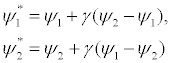(14)

Where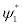and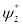are the muscular activations in the coupled nephron system.

To investigate the mode distribution in our system of two coupled nonidentical nephrons we again make use of the Poincar´e section technique to obtain charts of dynamical regions for the slow modes in the two nephrons. For this calculation we use the conditions Pt1=1:73 kPa and Pt2=1:73 kPa, respectively.

Figure 3 shows the distributions of TGF-mediated modes as plotted in the parameter plane defined by the coupling constant and the flow delay T2 for the second nephron. The flow delay for the first nephron is set to be T1 = 14 s. This implies that the natural period of the slow oscillations for this nephron is fixed (at about 33 s). The two feedback gain factors are set to be α1=9 and α1=12, respectively. With its higher gain factor, the amplitude of the slow oscillations in the second nephron is expected to exceed the amplitude of the internally generated slow oscillations in the first nephron (Figure 2).

Figure 3: (Online version in color.) Charts of TGF-mediated oscillations for the (a) weakly and (b) strongly oscillating nephron in the coupled system. White denotes quasiperiodic or chaotic dynamics, and light gray (green online) represents regular period-1 dynamics. In the region denoted 2, the weakly oscillating nephron completes two slow oscillations per full period of the strongly oscillating nephron. TOD denotes extinction of oscillatory dynamics, or “Total Oscillator Death”.

Inspection of Figure 3 reveals a number of interesting points:

(i) For values of the coupling parameter below approximately 0.15, the mode distributions for the weakly oscillating (Figure 3a) and the strongly oscillating (Figure 3(b)) nephron both display resonance dynamics near T2=14 s (=T1) and near T2=33 s. At the lower resonance, the two nephrons have approximately the same delay in their Henle flows and, hence, before coupling, nearly the same period of their slow TGF mediated oscillations. This produces 1:1 synchronization. At the higher resonance (T2=33 s), the period of the strongly oscillating nephron is approximately twice the period of the weakly oscillating nephron, and the coupled system attains a form of 2:1 entrainment between the two nephrons.

(ii) For higher values of the coupling parameter, the strongly oscillating nephron maintains its slow TGF-mediated oscillation both in a triangular region above the 1:1 synchronization region and in a broad band of coupling parameters (0:15< γ <0:31) that extends horizontally to the highest values of T2 investigated. The existence of this band is a main signature of “broadband synchronization”. In this band, the internally generated TGF-mediated oscillations of the weakly oscillating nephron are suppressed, and the nephron is entrained to follow the forcing from the strongly oscillating nephron.

(iii) The mode distribution for the weakly oscillating nephron (Figure 3a) displays a clearly pronounced 2:1 entrainment in the region around T2=33 s. The existence of this region is another characteristic feature of broadband synchronization. The region is delineated to the quasi periodic regime by a torus birth bifurcation, but the transition between the 1:1 and 2:1 entrainment zones in the region of broadband synchronization does not involve any bifurcation.

(iv) Above the region of broadband synchronization (and away from the 1:1 synchronization regime), the system displays total oscillator death (TOD), i.e., extinction of the dynamics of both nephrons. We also observe a small disk-shaped region between the 1:1 entrainment regime and the region of broadband synchronization where the system displays quasiperiodicity with different modes of synchronization (Figure 3).

The phase plots in Figure 4 illustrate the form and size of the oscillations observed in the two nephrons for different values of the bifurcation parameters and T2. The corresponding points are indicated on the chart of dynamical modes in Figure 4a. Other parameters are as before T1=14 s, α1=9, and α2=12.

Figure 4: (Online version in color.) (a) Chart of dynamical modes constructed for the slow mode oscillations of the weakly oscillating nephron in the coupled nephron model. (b)–(i) Phase plots for the two nephrons obtained with different combinations of the delay time T2 and the coupling parameter γ. Other parameters are T1 = 14 s, α1 = 9, and α2 = 12. Fig. (b)–(i) refer to points b–i in Fig. (a). The weakly oscillating nephron is to the left.

When operating in the triangular 1:1 entrainment regime, the phase space trajectories for the two nephrons are nearly identical both in shape and size (Figure 4b).

In the region of broadband synchronization (Figure 4c-e) immediately to the right of the small disk-shaped region of quasi periodicity, the two nephrons continue to oscillate in a 1:1 mode with the first nephron being driven by the second nephron and with a clear difference in oscillation amplitude. In Figure 4(e) we note the emergence of a small loop on the phase plot of the first nephron. This is related to the gradual transition from period-1 to period-2 dynamics of the weakly oscillating nephron. With further increase of the delay time T2 the point of operation moves into the large 2:1 region of broadband synchronization. Here, the first nephron continues to be driven by the second nephron. However, now in a 2:1 relation such that the first nephron completes two cycles each time the second nephron completes a single cycle (Figure 4f).

At the points g and h in the white area below the region of broadband synchronization the two nephrons oscillate autonomously, and the coupled system exhibits quasiperiodic (or synchronized periodic) dynamics. With its higher feedback gain, the second nephron generally shows the strongest oscillations. However, subjected to the significant forcing from this nephron, the weakly oscillating nephron displays the more irregular dynamics. To support the above interpretation we have used continuation techniques  to perform a more detailed two-dimensional bifurcation analysis of the coupled nephron system. Continuation allows one to follow a particular solution under variation of one or more parameters, to determine the points of bifurcation, and to follow bifurcation curves in parameter space. Figure 5 shows the results of such an analysis. As in Figure 3, T1=14 s, α1=9, and α2=12. For low coupling strengths we observe the narrow 1:1, 2:3, 3:5, 1:2, etc., synchronization tongues bounded by saddle-node bifurcations along their sides and connected via the torus bifurcation curve Tb. Along this curve the autonomous oscillations of the weakly oscillating nephron become suppressed by the forcing of the stronger nephron. Hence, quasiperiodic and synchronized periodic motions cease to exist, and for higher values of the coupling parameter, the weakly oscillating nephron operates as a stable equilibrium system under the forcing of the strongly oscillating nephron.

Figure 5: (Online version in color.) Two-dimensional bifurcation diagram for the coupled nephron model in a parameter plane spanned by the flow delay T2 of the second nephron and the coupling parameter γ. T1 = 14 s, α 1 = 9, and α 2 = 12.

This part of the diagram is in full accordance with previously cited results for externally forced chemical and biochemical oscillators [5-7]. It is interesting, however, to note that the regions of 1:1 and 1:2 synchronization, as bounded by the triangular saddle-node bifurcation structures SNa and SNb, are quite small. In these regions, both nephrons maintain their autonomous oscillations and synchronization occurs through the mutual adjustments of the two periodicities. For coupling strengths above the triangular regions, the autonomous oscillations of the weaker nephron are suppressed, and rather than a torus with coexisting periodic node and saddle solutions around an unstable focus, the system only displays a single periodic orbit. For even higher coupling strengths and for stronger mismatch between the natural frequencies, the region of broadband synchronization is delineated by the Hopf bifurcation curves Ha and Hb, respectively. Above Ha and to the right of Hb, the coupled nephron system displays a stable equilibrium point representing the state of total oscillator death. The existence of such astate appears first to have been suggested by Aronson et al. . Taylor and Kevrekidis [6,7] performed a preliminary bifurcation analysis of the transition to extinction, showing, in particular, how a small disk like region with quasiperiodicity and different synchronization regimes may develop from the point of intersection between Ha and Hb. In Figure 5, this region is delineated by the torus bifurcation curve Ta.

From their point of intersection, the two Hopf bifurcation curves continue as curves of unstable Hopf bifurcations. Crossing, for instance, the stable branch of the Hopf bifurcation curve Ha with decreasing values of the internephron coupling, a stable limit cycle is born as the equilibrium point turns into an unstable focus. With further reduction of the coupling parameter, as the point of operation crosses the unstable branch of Hb, the unstable focus equilibrium point destabilizes again (in a different sub-space), thus becoming a four times unstable focus with two pairs of complex conjugated eigenvalues in the positive halfplane. The structure delineated by the saddlenode bifurcation curve SNc represents a region in which solutions are folded over one another. In the lower part of this region we have two stable tori one beside the other and with the inset to an unstable torus providing the boundary of the two basins of attraction.

#### Role of differences in oscillator strength

To further illustrate the form of dynamics observed in the region of broadband synchronization, Figure 6 displays phase plots and characteristic temporal dynamics for the weakly (Figure 6 a) and strongly (Figure 6b) oscillating nephrons before coupling and for the same nephrons after coupling is introduced (Figure 6c). Here, α1=9 and T1=14 s for the weak nephron while α2=12 and T2=37:7 s for the strong nephron. The coupling parameter is γ2. These conditions correspond to a point near the middle of the region denoted 2 in Figure 3b, i.e., above the triangular region of 2:1 synchronization in Figure 5. It is obviously difficult to distinguish the state of 2:1 broadband synchronization as revealed in Figure 6 from a proper state of 2:1 synchronization. However, as mentioned above, there is no bifurcation involved in the transition between the 2:1 and 1:1 modes in the regime of broadband synchronization.

Figure 6: Phase plots and time series for: (a) single nephron for α = 9 and T = 14 s; (b) single nephron for α = 12 and T = 37:7 s; (c) first and second nephron for α 1 = 9, T1 = 14 s, α 2 = 12, T1 = 37:7 s and = 0:2. 2:1 entrainment between the weakly and the strongly oscillating nephron.

As argued in the Introduction, the appearance of broadband synchronization hinges directly on the lack of symmetry between the two nephrons, i.e., the difference between their feedback gain factors. This difference allows the dynamics of the second nephron to dominate that of the first nephron. When the coupling becomes strong enough for the second nephron to suppress the autonomous dynamics of the first nephron, the second nephron can still main tain its activity and excite oscillations in the first nephron. However, driving the oscillations of the first nephron inflicts additional damping on the second nephron and, when the coupling becomes strong enough to completely exhaust this nephron, the system suffers a transition to the region of total oscillator death (TOD).

The oscillatory strength of the individual nephron (and the amplitude of the limit cycle it generates) depends on a variety of parameters in the model, including, besides the feedback gain factor , the time delay associated with the flow through the loop of Henle, the flow resistance in this loop, the elastic compliance of the proximal tubule, etc. The width of the region of broadband synchronization in this way depends on the net result of different parameters for the two nephrons.

To illustrate this point, Figure 7 shows the peak-to-peak variation R for the observed oscillations in the normalized arteriolar radius r as a function of the delay time T in the loop of Henle for gain factors of α=9 and α=12. R10 is the peak-to-peak variation of the arteriolar radius for α=9 and T=T1=14 s. We notice that a gain factor of α=12 produces a significant larger oscillation amplitude for all considered values of T. Moreover, for α=12, the value of R for large values of T appears to stabilize around the value R20. This provides the background for postulating that the region of broadband synchronization (as observed for coupled van der Pol oscillators [9-11]) may continue to exist even for very large values of T2.

Figure 7: Peak-to-peak variations R in the normalized arteriolar radius as function of the feedback delay for different feedback gain factors.

As already emphasized, the appearance of broadband synchronization is related to a lack of balance in the autonomous dynamics of the two nephrons. Hence, if we choose a set of parameters T1, 1, and 2 for which the difference (R20-R10) between the peak-topeak variations of the normalized arteriolar radii for the two nephrons is small, then the phenomenon of broadband synchronization should become less pronounced or, perhaps, even disappear.

Figure 8 illustrates the first possibility. Here, we have plotted the peak-topeak values R1 and R2 of the normalized radii r1 and r2 as functions of the coupling parameter for two different sets of the parameters T1, α1, and α2. In both plots we note that the amplitude of the oscillations is smaller for the first nephron than for the second nephron.

Figure 8: Variation of the oscillator amplitudes through the region of broadband synchronization. Peak-to-peak values R1 and R2 for the variations in the arteriolar radii r1 and r2 as functions of the coupling parameter for T1 = 14 s and α 1 = 9. (a) T2 = 28 s and α2 = 12, (b) T2 = 29 s and α 2 = 14. γ1 represents the lower boundary of the region of broadband synchronization, and γ2 is the upper boundary.

More importantly, however, there is a characteristic structure in the variation of R1 and R2, such that R1 exhibits a sharp drop in the interval immediately before 1, the value of the coupling parameter at which broadband synchronization sets in. Moreover, both R1 and R2 drop to zero at γ2, the value of the coupling parameter at which the region of broadband synchronization terminates. Hence, we conclude that the amplitude of the weaker oscillator drops markedly just before broadband synchronization sets in, and that a transition from broadband synchronization occurs as the system enters the region of total oscillator death at γ2, when the amplitudes of both oscillators drop to zero.

So far our examples have considered the coupling of ephrons with a fairly large difference in their gain factors. With α1=9, the first nephron operates relatively close to the threshold of oscillations, and the limit cycle it produces is weak and small in amplitude. With α2=12 (or even 14), on the other hand, the second nephron produces TGF-mediated oscillations that easily dominate the dynamics of the first nephron.

To stress the significance of such a pronounced difference in gain factors (or oscillation amplitudes), Figure 9 shows a chart of dynamical modes calculated for α1=10 and α2=12. The delay time of the first nephron remains unchanged at T1=14 s. In spite of the finite difference in gain factors, however, a regime of broadband synchronization does not develop. The two oscillators are now too equal in strength and, for large mistuning; the system displays a transition from quasiperiodicity to total oscillator death through a pair of nearly coinciding torus and Hopf bifurcations. As this transition is followed for increasing coupling parameters, the system first undergoes an inverse torus bifurcation as the stronger oscillator suppresses the weaker oscillator. However, as this process transfers additional losses (dissipation) to the stronger oscillator, this oscillator also stops to oscillate, now through an inverse Hopf bifurcation.

Figure 9: (Online version in color.) Chart of dynamical modes constructed for the slow mode of the first nephron with T1 = 14 s, α1 = 10, and α2 = 12. With these values of α the nephrons are too similar for an actual region of broadband synchronization to develop.

#### Conclusion

Discussions of physiological synchronization processes typically take their point of departure in the classic picture of Arnol’d tongues bounded on either side by saddle-node bifurcation curves at which two-frequency quasiperiodicity is replaced by resonance dynamics in the form of a pair of node and saddle cycles [1,2]. This picture is justified as long as the coupling is relatively weak.

A different, and less studied, situation arises in the case where the interacting subsystems display a relatively strong coupling in connection with a significant difference in oscillator strength. In the present paper we have used a previously developed model of a pair of interacting nephrons (functional units of the kidney) to illustrate a number of significant phenomena that are generic to this situation. The best known aspect of this problem is the transition between the state of 1:1 synchronization and the state in which the stronger oscillator suppresses the natural dynamics of the other. This transition is discussed in standard textbooks on synchronization [1,2]. However, this discussion fails to emphasize how small the synchronization regions often are and how difficult it can be to distinguish between synchronization and oscillator suppression on the basis of experimental observations. In computer simulations where the parameters can be varied continuously the transition from quasiperiodicity to synchronization can be distinguished from the transition from quasiperiodicity by means of the involved bifurcations. Although this distinction may be somewhat qualitative, one can also follow the development of the frequency spectrum. The transition from quasiperiodicity to synchronization involves the mutual adjustment of the two frequencies, and the spectrum shows a gradual approach of the corresponding peaks as the coupling increases. The transition from quasiperiodicity to oscillator suppression, on the other hand, involves the gradual reduction and final disappearance of the peak associated with the weaker oscillator.

A more detailed discussion of the transition from synchronization in the low coupling regime to oscillator suppression in the high coupling regime was presented in a series of papers in the chemical literature already in the 1990’s [4-6]. These papers clearly emphasized the generic nature of the developed theory. However, in spite of the large number of different experiments conducted on forced and coupled physiological oscillator systems, we are only aware of a single analysis that consider the effects of a transition to oscillator suppression . In our view there is a significant risk that many studies of forced (or coupled) physiological oscillators are actually conducted under conditions where the external forcing (or the stronger oscillator) completely suppresses the natural oscillation of the physiological system (the weaker oscillator). The transition from the suppressed state to total oscillator death was examined by a few authors in the chemical literature [7,8], and the transition from synchronized to suppressed oscillations has also been investigated for a spatially extended microwave oscillator . In the present paper we have renewed attention to this problem by illustrating the difficulties associated with a distinction between synchronized and suppressed dynamics. We have also determined the size and form of the regime of broadband synchronization (oscillator suppression), and we have examined the internal structure of this regime. A preliminary version of this paper (in Russian) has appeared in Rus. J. Nonlin. Dyn. 8 (2012) 875-896.

#### Acknowledgement

E.M. acknowledges support from the Danish Medicines Agency (DKMA). A. P. K. and Yu. P. E. Acknowledge partial support from the Presidents of Russian Federation Grant for support of leading schools of sciences No. NSh- 1726.2014.2. Yu.P.E. also acknowledges support from the Russian Foundation for Basic Research Grant No. 12–02–31493.

#### References

Select your language of interest to view the total content in your interested language

### Article Usage

• Total views: 12634
• [From(publication date):
May-2014 - Nov 15, 2019]
• Breakdown by view type
• HTML page views : 8801Can't read the image? click here to refresh